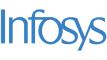New update is available. Click here to update.
Last Updated: 20 Nov, 2020

# 3Sum

Moderate+58 more companies

## Problem statement#### An array is said to have a triplet {ARR[i], ARR[j], ARR[k]} with sum = 'K' if there exists three indices i, j and k such that i!=j, j!=k and i!=j and ARR[i] + ARR[j] + ARR[k] = 'K'.

##### Note:
``````1. You can return the list of values in any order. For example, if a valid triplet is {1, 2, -3}, then {2, -3, 1}, {-3, 2, 1} etc is also valid triplet. Also, the ordering of different triplets can be random i.e if there are more than one valid triplets, you can return them in any order.
2. The elements in the array need not be distinct.
3. If no such triplet is present in the array, then return an empty list, and the output printed for such a test case will be "-1".
``````
##### Input Format:
``````The first line of the input contains an integer T, denoting the number of test cases.

The first line of each test case contains the integer N, denoting the size of the array.

The second line of each test case contains N space-separated integers denoting the array elements.

The third line of each test case contains the integer K, denoting the required sum for each triplet.
``````
##### Output Format:
``````For each test case, every line of output contains three spaced integers denoting a valid triplet as described in the statement. Refer to sample input 2 for more clarification.
``````
##### Note :
``````You do not need to print anything, it has already been taken care of. Just implement the given function.
``````
##### Constraints:
``````1 <= T <=  50
1 <= N <= 10^3
-10^6 <= ARR[i] <= 10^6
-10^9 <= K <= 10^9

Time Limit: 1 sec
``````## Approaches

### 01 Approach• The most trivial approach would be to find all triplets of the array and count all such triplets whose ‘SUM’ = 'K'.
• We can find the answer using three nested loops for three different indexes and check if the values at those indexes sum up to 'K'.
• Create a set  to keep the track of triplets we have visited. Run first loop from i = 0 to i = ‘N’ - 3, second loop from j = i + 1 to j = ‘N’ - 2 and third loop from ‘K’ = j + 1 to ‘K’ = ‘N’ - 1.
• Check if ‘ARR[i]’ + ‘ARR[j]’ + ‘ARR[K]’ = ‘K’
• If the triplet is not present in the set, then print the triplet and insert the triplet into the set since we need distinct triplets only.
• Else continue.